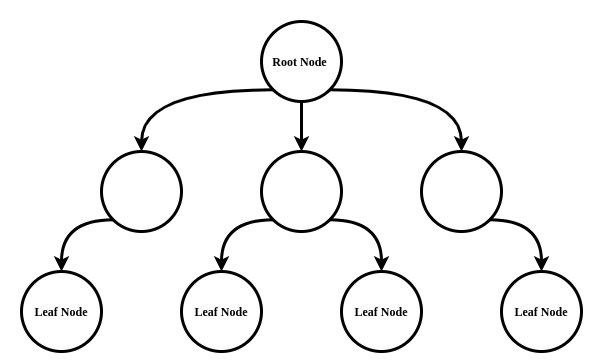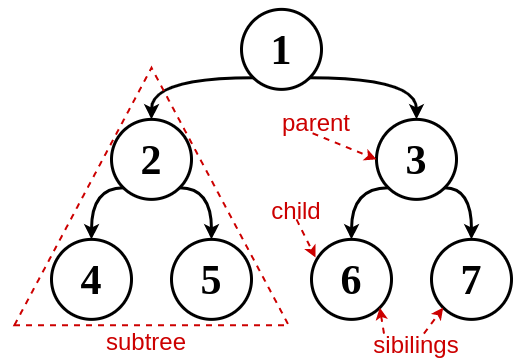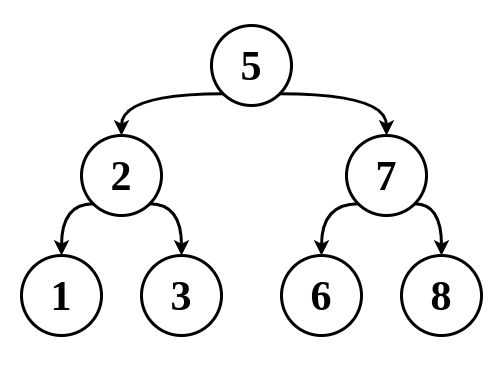# Ricardo Borges

Personal blog## Data Structures in Typescript - Binary Search Tree

### What is a Tree data structure

Before we talk BST, we have to understand that a tree is a kind of Graph with a root node and no cycles, each node can have zero or more child nodes, nodes can store any type of data, also those nodes may or may not be in a particular order, nodes that have no children are called leaves.### Binary Tree

A Binary tree is a tree that each node has up to two nodes:### Binary Search Tree

A BST is a Binary tree that follows these properties:

• All left descendants nodes are less or equal to their parent node
• All right descendants nodes are greater than their parent nodeBST definitions can slightly vary, you may find a BSTs that the left subtree is less than the parent node (`left subtree < parent <= right subtree`).

Also, some BSTs may or may not allow duplicated nodes.

BST is useful when you need to insert, delete and search comparable elements. You can use an array for those operations, but, although access an element in an array is done in a constant time, whenever you insert or delete an element, the other elements have to be shifted, in a BST, on the other hand, you only need to adjust the pointers.

### Searching in the BST

The search starts from the root node, if the element you are searching for is greater than the current node value, then search for it in the right subtree, otherwise search in the left subtree, repeat this until the current node value is equal to the element you are searching for or until you reach a leaf node and there is nowhere to go:

``````1search(data) {
2    // empty tree
3    if (!root) return;
4
5    // start from root node
6    let current = root;
7
8    while (current.data !== data) {
9        // data greater than current element
10        if (data > current.data) {
11
12        // you are on a leaf node, nowhere to go
13        if (!current.rightNode) return;
14
15        // go to the right subtree
16        current = current.rightNode;
17        } else {
18
19        // you are on a leaf node, nowhere to go
20        if (!current.leftNode) return;
21
22        // go to the left subtree
23        current = current.leftNode;
24        }
25    }
26
27    return current;
28}
``````

### Inserting in the BST

When inserting a new element in BST you have to keep its properties, so start from the root node, if the new element value is greater than the root node value, search for an empty position in the right subtree, otherwise search for an empty position in the left subtree:

``````1insert(data) {
2  // empty tree, insert the first node (the root node)
3  if (!root) {
4    root = new Node(data);
5    return root;
6  }
7
8  // start from the root node
9  let current = root;
10
11  while (true) {
12    if (data > current.data) {
13      // search for an empty position in the right subtree
14      if (current.rightNode) {
15        current = current.rightNode;
16      } else {
17        // insert node
18        current.rightNode = new Node(data);
19        return current.rightNode;
20      }
21    } else {
22      // search for an empty position in the left subtree
23      if (current.leftNode) {
24        current = current.leftNode;
25      } else {
26        // insert node
27        current.leftNode = new Node(data);
28        return current.leftNode;
29      }
30    }
31  }
32}
``````

### Binary Search Tree traversal

There are three ways to traverse a BST, they differ on which order the nodes are visited (often, printed):

#### In-Order traversal

First visit left branch, then the current node, and finally the right branch, because of how elements are distributed in the BST, they will be visited in the ascending order:

``````1inOrderTraversal(node) {
2  if (node) {
3    inOrderTraversal(node.leftNode);
4    console.log(node.data);
5    inOrderTraversal(node.rightNode);
6  }
7}
``````

#### Pre-Order traversal

Visit the current node before its children:

``````1preOrderTraversal(node) {
2  if (node) {
3    console.log(node.data);
4    this.preOrderTraversal(node.leftNode);
5    this.preOrderTraversal(node.rightNode);
6  }
7}
``````

#### Post-Order traversal

Visit the current node's children first, then the current node:

``````1postOrderTraversal(node) {
2  if (node) {
3    this.postOrderTraversal(node.leftNode);
4    this.postOrderTraversal(node.rightNode);
5    console.log(node.data);
6  }
7}
``````

Here's an implementation of a BST in typescript:

``````1export class BinarySearchTreeNode<T> {
2  data: T;
3  leftNode?: BinarySearchTreeNode<T>;
4  rightNode?: BinarySearchTreeNode<T>;
5
6  constructor(data: T) {
7    this.data = data;
8  }
9}
10
11export class BinarySearchTree<T> {
12  root?: BinarySearchTreeNode<T>;
13  comparator: (a: T, b: T) => number;
14
15  constructor(comparator: (a: T, b: T) => number) {
16    this.comparator = comparator;
17  }
18
19  insert(data: T): BinarySearchTreeNode<T> | undefined {
20    if (!this.root) {
21      this.root = new BinarySearchTreeNode(data);
22      return this.root;
23    }
24
25    let current = this.root;
26
27    while (true) {
28      if (this.comparator(data, current.data) === 1) {
29        if (current.rightNode) {
30          current = current.rightNode;
31        } else {
32          current.rightNode = new BinarySearchTreeNode(data);
33          return current.rightNode;
34        }
35      } else {
36        if (current.leftNode) {
37          current = current.leftNode;
38        } else {
39          current.leftNode = new BinarySearchTreeNode(data);
40          return current.leftNode;
41        }
42      }
43    }
44  }
45
46  search(data: T): BinarySearchTreeNode<T> | undefined {
47    if (!this.root) return undefined;
48
49    let current = this.root;
50
51    while (this.comparator(data, current.data) !== 0) {
52      if (this.comparator(data, current.data) === 1) {
53        if (!current.rightNode) return;
54
55        current = current.rightNode;
56      } else {
57        if (!current.leftNode) return;
58
59        current = current.leftNode;
60      }
61    }
62
63    return current;
64  }
65
66  inOrderTraversal(node: BinarySearchTreeNode<T> | undefined): void {
67    if (node) {
68      this.inOrderTraversal(node.leftNode);
69      console.log(node.data);
70      this.inOrderTraversal(node.rightNode);
71    }
72  }
73
74  preOrderTraversal(node: BinarySearchTreeNode<T> | undefined): void {
75    if (node) {
76      console.log(node.data);
77      this.preOrderTraversal(node.leftNode);
78      this.preOrderTraversal(node.rightNode);
79    }
80  }
81
82  postOrderTraversal(node: BinarySearchTreeNode<T> | undefined): void {
83    if (node) {
84      this.postOrderTraversal(node.leftNode);
85      this.postOrderTraversal(node.rightNode);
86      console.log(node.data);
87    }
88  }
89}
90
91function comparator(a: number, b: number) {
92  if (a < b) return -1;
93
94  if (a > b) return 1;
95
96  return 0;
97}
98
99const bst = new BinarySearchTree(comparator);
100
101bst.insert(5);
102
103bst.insert(2);
104bst.insert(3);
105bst.insert(1);
106
107bst.insert(7);
108bst.insert(6);
109bst.insert(8);
110
111bst.inOrderTraversal(bst.root);
``````

Unlike Graphs, a tree doesn't really need a Tree class, just a Node class would usually suffice.